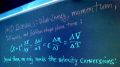# UM-StL Physics 112 - Trip to Andromeda

The problem is this: You board a spaceship that accelerates at 1 "gee" continuously until it has traveled half of the 2.2 million lightyear distance to the Andromeda Galaxy*. It then decelerates (also at 1 "gee") over the remaining 1.1 million lightyears of distance to halt in a star system with an earth-like planet orbiting a sol-like star in the Andromeda galaxy itself! How much older are you at the end of the trip? You may be surprised.

Notes below outline (but do not complete) a novel solution to this problem that makes use of Galilean chase-plane time T, and Galilean-kinematic velocity V = dx/dT. These obey Galileo's equations for constant (proper) acceleration at any speed, even the classical expression for kinetic energy K=½mV2. A solution in terms of the more widely used (and useful) coordinate-velocity v = dx/dt may instead be found here. In both cases, we also find it useful to define from Minkowski's spacetime Pythagoras' theorem, i.e. the metric equation: (c dτ)2 = (c dt)2 - (dx)2, the proper or traveler time τ and the proper velocity w = dx/dτ.

• The "one map two-three clock" equations of anyspeed acceleration are summarized here:.
• Intro to the variables used here: this PDF or pages: 1,2,3,4.
• Related notes on anyspeed modeling, modernizing Newton, and one-map two-clocks
• An April 2005 "live remote" platform for empirical study of the spacetime connection.
• Related puzzlers are here and here. Versions with answers are here and here.
• Galilean-kinematic: {chase-plane time, velocity}={T, V},
• Map-kinematic: {map time, coordinate-velocity}={t, v}, and
• Traveler-kinematic: proper-{time, velocity, acceleration}={τ, w, α}.
• If Andromeda is too far away, consider a race to Alpha-Centauri!
Background: Because of the high speeds involved, only clocks in Galileo's equation-saving "chase plane" follow the time parameter T used in Galileo's classical acceleration equations. Instead, the rocket or "proper-time" dτ elapsed for you on each of the two constant acceleration legs of the trip is

dτ=(ArcSinh[wf/c] - ArcSinh[wo/c])*c/α,

where the speed of light c is 3x10^8[m/s2] or 1[ly/yr], proper acceleration α is 9.8[m/s2] or 1.03[ly/yr2], and wf & wo denote final & initial proper velocities (w = dx/dτ) in units of "distance traveled per unit of traveler time". Relativists will recognize proper velocity w as c Sqrt[γ2-1], where γ = E/mc2. Proper velocities here can be figured by the conversion

w = V Sqrt[1 + ¼( V/c )2],

from "Galilean velocities" Vf and Vo which obey the classical equations for constant acceleration. The standard equation

Vf2 - Vo2 = 2 α dx

(where dx is distance traveled) in particular should do the job. Given initial/final proper velocities, the maptime dt elapsed is simply (wf-wo)/α, and coordinate velocity v=dx/dt is w/γ. Can you show that finite proper velocities w require that v is always less than c?

Note: The "Galilean velocity" V is the velocity familiar from introductory physics books extended to all velocities as Galileo might have presumed through the kinetic energy equation K = (1/2)mV2. It thus becomes the derivative of x with respect to a "Galilean chase-plane time" T which can be used to track events during 1D acceleration, but which at high speed follows neither traveler nor earth based clocks. At high speeds Galilean velocity V is not the Lorentz/Minkowski coordinate velocity v = dx/dt.

* The Andromeda Galaxy is one of the most distant objects visible to the naked eye. Total distance traveled is 2.2x106 [ly] x 9.46x1015 [m/ly] = 2.08x1022 [meters].

Followup: Do you like doing relativistic kinematics in this way? More examples now under construction, along with some answers, will be put here.
• Copyright (1970-2005) by Phil Fraundorf
• Dept. of Physics & Astronomy, University of Missouri-StL, St. Louis MO 63121-4499
• Phone: (314)516-5044, Fax:(314)516-6152
• For source, cite URL at http://www.umsl.edu/~fraundor/andromed.html
• Version release date: 30 April 2005.
• Through July '96, this and a mirror page on www.umsl.edu had received 367 visits.
• Since 1 Aug 1996, you are visitor number [broken counter].
• Mindquilts site page requests ~2000/day approaching a million per year.
• Requests for a "stat-counter linked subset of pages" since 7 April 2005: .

• Send comments, your answers to problems posed, and/or complaints, to philf@newton.umsl.edu. This page contains original material, so if you choose to echo in your work, in print, or on the web, a citation would be cool. ` (Thanks. /philf :)`